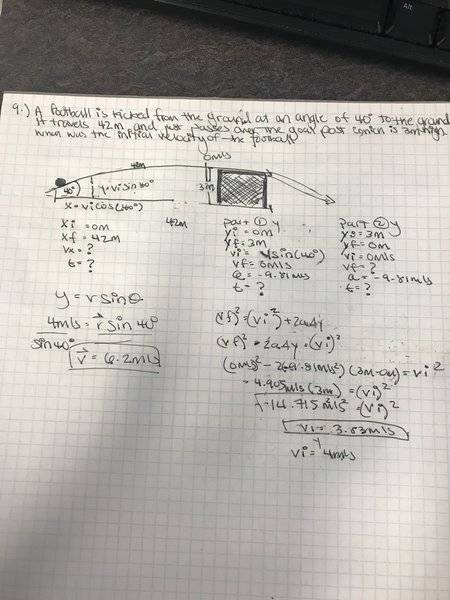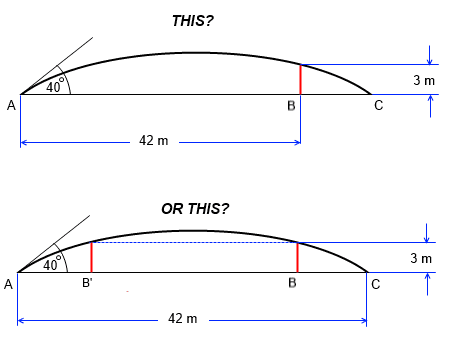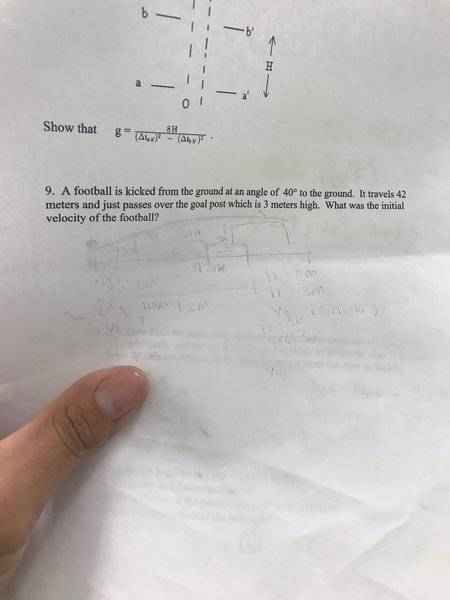# Projectile motion HW check

## Homework Statement

A football is kicked from the ground at an angle of 40° to the ground. It travels 42m and just passes over the goal post which is 3m high. What was the inital velocity of the football?

## Homework Equations

vfinal2 = vinitial2 +2a(yfinal-yinitial)

## The Attempt at a Solution

Assuming that the football from the start to finish creates a symmetric parabolic line, I realize that when the football reaches it's highest point, it stops gaining velocity and has an acceleration of -9.81m/s2. With that information, I set yinitial = 0m and yfinal = 3m. From there, I used the equation above to find vinitial (rearranging the formula to have vinitial2 = vfinal2-2a(yfinal-yinitial) , (numbers plugged in.. vinitial2 = (0m/s)2 -2(-9.81m/s2)(3m-0m)) and got vinitial = 4m/s in the y direction. Knowing that velocity in the y direction = velocity(sin(Θ)), rearranging the function to yvelocity/(sin(Θ)) = velocity, I got 6.2m/s as initial velocity of the football. I appreciate any feedback.Picture of my work

rude man
Homework Helper
Gold Member
Where did you include the total travel distance of 42m?
I deleted my first post since I worked the problem with 42m travel and then realized I had not invoked the 3m goal posts height! So - my hint is that you can combine 42m length and 3 m height if you (1) don't assume that 3m is the highest the ball will ever go, and (2) that you realize that you have an extra independent variable, to wit, the distance between where the football is kicked and the goal posts.

Last edited:
Where did you include the total travel distance of 42m?
It's not included anywhere since I just mainly used y components to find the velocity.

rude man
Homework Helper
Gold Member
It's not included anywhere since I just mainly used y components to find the velocity.
Well, did you calculate your total distance? Wanna bet it's not 42m? It's part of your problem to solve, right?

Well, did you calculate your total distance? Wanna bet it's not 42m?
I thought the total distance was 42m (in the x-direction)?..

rude man
Homework Helper
Gold Member
I thought the total distance was 42m (in the x-direction)?..
You never calculated it, did you? Given what you did, i.e. use the angle of kick and the goal post height, do you then get a distance of 42m? And if not, did you do the problem as stated?
Again, consider that the highest the ball went might be higher than the goal post height, either before or after the ball traverses the goal post plane.

You never calculated it, did you? Given what you did, i.e. use the angle of kick and the goal post height, do you then get a distance of 42m? And if not, did you do the problem as stated?
Again, consider that the highest the ball went might be higher than the goal post height, either before or after the ball traverses the goal post plane.

When you say to use the goal post height and the angle of kick, finding the hypotenuse of the right triangle (assuming that the ball goes in a diagonal line)?

rude man
Homework Helper
Gold Member
If you assume 42m distance you get max football height at 42/2 = 21 m from the kicking spot, right? With that you can compute what v has to be in order for the ball to travel 42m. Now calculate how high the ball is when it clears the goal posts. Is it 3m? More? If more then it didn't JUST clear the goal posts did it?
When you say to use the goal post height and the angle of kick, finding the hypotenuse of the right triangle (assuming that the ball goes in a diagonal line)?
?? Your locus is a parabola, not a straight line ...
This is a bit of a tricky problem in that it involves an extra dimension which is the distance between where the football is spotted and the goal posts. They don't ask you for that distance (haven't computed it myself yet; there are two possible distances: one is where the ball clears the posts BEFORE it reaches max height, the other where it reaches max height AFTER it just cleared the posts) but just realize that you can move the kick point anywhere you choose long as the ball (1) travels 42m total and (2) JUST clears the 3m goal posts. They could have made the problem even harder had they asked you for those extra distances!

Last edited:
If you assume 42m distance you get max football height at 42/2 = 21 m from the kicking spot, right? With that you can compute what v has to be in order for the ball to travel 42m. Now calculate how high the ball is when it clears the goal posts. Is it 3m? More? If more then it didn't JUST clear the goal posts did it?

Wow, that completely slipped my mind. I wouldn't think the height of the ball reaches 3m since that's the height of the goal post, it would actually hit it if that were the case. How would I got about finding the correct height? I hope it's not something arbitrary..

rude man
Homework Helper
Gold Member
Wow, that completely slipped my mind. I wouldn't think the height of the ball reaches 3m since that's the height of the goal post, it would actually hit it if that were the case. How would I got about finding the correct height? I hope it's not something arbitrary..
OK, this may be my last post on this, I will try to summarize the situation but you have to provide think power too!
1. Compute v to get a total distance of 42m. This is actully the answer to the problem, but you won't learn nearly as much if you leave it at that.
2. So how about you compute the max. height the ball would reach. I think you'll find that that's quite a bit higher than 3m.
3. So, here's the fun part, and it is not that hard: what do you have to do to make the ball just barely clear the goal posts? Draw the parabola with 42m distance and the compute max. height at the top, then put marks at 3m height on the parabola, then what would you do to just clear the goal posts?
(I've given you a hint before as to what you might want to do.)

I think I found my answer but I'm not proud of it because I used parts of what someone else did on chegg.com but I understand the method.

velocity in the x-direction:
x = v(t)
substitution x = (vcos40°)t
plugging in the total distance from the problem.. 42m = (vcos40°)t
bringing the t to the other side.. 42m/t = vcos40°
42m/t = 0.7660v (cos at 40° = 0.7760)
Now, dividing the constants gives me
v = 54.83/t

Now with that information known, I can use it to help me find t by using these numbers in the y-direction..
y = v0t + ½(a)t2
3m = (vsin40°)t + ½(a)t2..
now to substitute the v from the x-direction and cancelling out t's. (sin40° = .6427)
3m = (35.24m) -( 4.905 m/s2)t2
subtracting 35.24m to the other side..
-32.24m = -4.905m/s2(t2)
dividing the acceleration to the other side and cancelling out the meters..
6.57s = t2
t = 2.5s

Now to get velocity by plugging in t
v = (54.83m/2.5s)
v = 21 m/s

I would have never thought about doing it this way.Ray Vickson
Homework Helper
Dearly Missed
I think I found my answer but I'm not proud of it because I used parts of what someone else did on chegg.com but I understand the method.

velocity in the x-direction:
x = v(t)
substitution x = (vcos40°)t
plugging in the total distance from the problem.. 42m = (vcos40°)t
bringing the t to the other side.. 42m/t = vcos40°
42m/t = 0.7660v (cos at 40° = 0.7760)
Now, dividing the constants gives me
v = 54.83/t

Now with that information known, I can use it to help me fiwnd t by using these numbers in the y-direction..
y = v0t + ½(a)t2
3m = (vsin40°)t + ½(a)t2..
now to substitute the v from the x-direction and cancelling out t's. (sin40° = .6427)
3m = (35.24m) -( 4.905 m/s2)t2
subtracting 35.24m to the other side..
-32.24m = -4.905m/s2(t2)
dividing the acceleration to the other side and cancelling out the meters..
6.57s = t2
t = 2.5s

Now to get velocity by plugging in t
v = (54.83m/2.5s)
v = 21 m/s

I would have never thought about doing it this way.You are really doing it the hard way.

For initial horizontal and vertical velocities of ##v_x, v_y## we have ##x = v_x t## and ##y = v_y t - \frac{1}{2} g t^2##. We have ##y = 0## at ##t = 0## and at ##t = T_1 \equiv 2 v_y/g##. The football's arc starts from the ground at time ##t=0## and ends on the ground again at time ##t = T_1##. The horizontal travel distance is ##X = v_x T_1 = 2 v_x v_y/g##. Note that ##v_x = v_0 \cos(\theta)## and ##v_y = v_0 \sin(\theta)##, so ##X = (v_0^2/g) 2 \sin(\theta) \cos(\theta) = v_0^2 \sin(2 \theta)/g##. Thus, ##42 = v_0^2 \sin(80^o)/9.81,## giving ##v_0 = 20.4542 \;(m/s)##.

Note that nowhere did we need to use anything about "clearing the goal post at height 3m", but now that we know ##v_x## and ##v_y## we can easily enough figure out where that would occur (at the time ##t## that solves ##v_y t - \frac{1}{2} g t^2 = 3##).

Last edited:
•HRubss
rude man
Homework Helper
Gold Member
I think I found my answer but I'm not proud of it because I used parts of what someone else did on chegg.com but I understand the method.

velocity in the x-direction:
x = v(t)
substitution x = (vcos40°)t
plugging in the total distance from the problem.. 42m = (vcos40°)t
bringing the t to the other side.. 42m/t = vcos40°
42m/t = 0.7660v (cos at 40° = 0.7760)
this t is total flight time
Now with that information known, I can use it to help me find t by using these numbers in the y-direction..
y = v0t + ½(a)t2
3m = (vsin40°)t + ½(a)t2..
This is time t to reach 3m. It is lower than the time of flight (also, there are two solutions for t here; you picked the longer one arbitrarily I guess).So this computation will give the wrong answer.

Your answers are not that far from right but unfortunately the method is wrong.
You are really doing it the hard way.
It isn't just the hard way, it's the wrong way. And should you be posting the entire solution anyway?

Ray Vickson
Homework Helper
Dearly Missed
this t is total flight timeThis is time t to reach 3m. It is lower than the time of flight (also, there are two solutions for t here; you picked the longer one arbitrarily I guess).So this computation will give the wrong answer.

Your answers are not that far from right but unfortunately the method is wrong.
It isn't just the hard way, it's the wrong way. And should you be posting the entire solution anyway?

Usually not, but he already posted a solution (that he copied from somebody else, he says).

rude man
Homework Helper
Gold Member
Usually not, but he already posted a solution (that he copied from somebody else, he says).
Which was totally wrong.

lewando
Homework Helper
Gold Member
Please, for my benefit, which problem is to be solved?If you assume 42m distance you get max football height at 42/2 = 21 m from the kicking spot, right?
Suggests the bottom problem.

rude man
Homework Helper
Gold Member
Please, for my benefit, which problem is to be solved?
View attachment 210816

Suggests the bottom problem.
Good point. The problem statement is a bit ambiguous. I assumed the bottom one as you do.

haruspex
Homework Helper
Gold Member
2020 Award
Good point. The problem statement is a bit ambiguous. I assumed the bottom one as you do.
If 42m is to landing on the ground then the information about clearing a bar at 3m cannot be relevant. We can fit the location of the bar to whatever trajectory we come up with, provided it rises to at least 3m.
Of course, the 3m might have been thrown in to provide an exercise in deciding which information is relevant, but more likely it is because the 42m is supposed to be the horizontal distance to the bar.
@HRubss, have you stated the question word for word?

If 42m is to landing on the ground then the information about clearing a bar at 3m cannot be relevant. We can fit the location of the bar to whatever trajectory we come up with, provided it rises to at least 3m.
Of course, the 3m might have been thrown in to provide an exercise in deciding which information is relevant, but more likely it is because the 42m is supposed to be the horizontal distance to the bar.
@HRubss, have you stated the question word for word?Yes, here's the worksheet.

You are really doing it the hard way.

For initial horizontal and vertical velocities of ##v_x, v_y## we have ##x = v_x t## and ##y = v_y t - \frac{1}{2} g t^2##. We have ##y = 0## at ##t = 0## and at ##t = T_1 \equiv 2 v_y/g##. The football's arc starts from the ground at time ##t=0## and ends on the ground again at time ##t = T_1##. The horizontal travel distance is ##X = v_x T_1 = 2 v_x v_y/g##. Note that ##v_x = v_0 \cos(\theta)## and ##v_y = v_0 \sin(\theta)##, so ##X = (v_0^2/g) 2 \sin(\theta) \cos(\theta) = v_0^2 \sin(2 \theta)/g##. Thus, ##42 = v_0^2 \sin(80^o)/9.81,## giving ##v_0 = 20.4542 \;(m/s)##.

Note that nowhere did we need to use anything about "clearing the goal post at height 3m", but now that we know ##v_x## and ##v_y## we can easily enough figure out where that would occur (at the time ##t## that solves ##v_y t - \frac{1}{2} g t^2 = 3##).

When my professor worked it out, he did the exact same thing. Question though, how did you get ##t = T_1 \equiv 2 v_y/g##?

When my professor worked it out, he did the exact same thing. Question though, how did you get ##t = T_1 \equiv 2 v_y/g##?
Nevermind, figured it out after reading part of my textbook. Thanks for the help guys.

SammyS
Staff Emeritus
Homework Helper
Gold Member
Please, for my benefit, which problem is to be solved?Suggests the bottom problem.
In American football, it is the usual case that you know the distance to the goal posts from the spot at which the ball is to be kicked.

Therefore, I suggest that the upper sketch may well represent the problem to be solved. It seems to me that this makes the problem only a bit more challenging.

In American football, it is the usual case that you know the distance to the goal posts from the spot at which the ball is to be kicked.

Therefore, I suggest that the upper sketch may well represent the problem to be solved. It seems to me that this makes the problem only a bit more challenging.
Though we used the range of 3m in the y direction, realistically, the ball would have hit the post and bounced off but we neglected that while we worked the problem out.